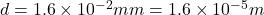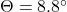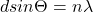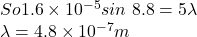Monochromatic (single-wavelength) light falling on two slits 0.016 mm apart produces the fifth-order bright spot at an 8.8◦angle. What is th

Question

Monochromatic (single-wavelength) light falling on two slits 0.016 mm apart produces the fifth-order bright spot at an 8.8◦angle. What is the wavelength of the light used?

in progress 0
5 months 2021-08-29T12:33:37+00:00 2 Answers 16 views 0

1. Given Information:

distance = d = 0.016 mm = 0.0016 cm = 0.000016 m

Angle = θ = 8.8°

bright spot = m = 5

Required Information:

Wavelength =  λ = ?

Wavelength = 4.89×10⁻⁷ m

Explanation:

Monochromatic light passes through a double slit. The corresponding diffraction is given by the equation:

dsinθ = mλ

where d is the distance between two slits, m is the order of the diffraction, θ is the angle and λ is the wavelength of the light wave.

λ = dsinθ/m

λ = 0.016*sin(8.8°)/5

λ = 4.89×10⁻⁷ m

Therefore, the wavelength of the monochromatic light falling on two slits is 4.89×10⁻⁷ m

the wavelength of the light used is 4.8 × 10⁻⁷m

Explanation:

We have given distance between the two slitsAngleWe know that for nth order fringeIn question it is given that 5th order so n =5